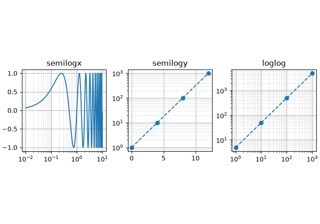# matplotlib.axes.Axes.loglog#

Axes.loglog(*args, **kwargs)[source]#

Make a plot with log scaling on both the x- and y-axis.

Call signatures:

```loglog([x], y, [fmt], data=None, **kwargs)
loglog([x], y, [fmt], [x2], y2, [fmt2], ..., **kwargs)
```

This is just a thin wrapper around `plot` which additionally changes both the x-axis and the y-axis to log scaling. All the concepts and parameters of plot can be used here as well.

The additional parameters base, subs and nonpositive control the x/y-axis properties. They are just forwarded to `Axes.set_xscale` and `Axes.set_yscale`. To use different properties on the x-axis and the y-axis, use e.g. `ax.set_xscale("log", base=10); ax.set_yscale("log", base=2)`.

Parameters:
basefloat, default: 10

Base of the logarithm.

subssequence, optional

The location of the minor ticks. If None, reasonable locations are automatically chosen depending on the number of decades in the plot. See `Axes.set_xscale`/`Axes.set_yscale` for details.

Non-positive values can be masked as invalid, or clipped to a very small positive number.

**kwargs

All parameters supported by `plot`.

Returns:
list of `Line2D`

Objects representing the plotted data.

## Examples using `matplotlib.axes.Axes.loglog`#Secondary Axis

Secondary AxisLog Demo

Log Demo